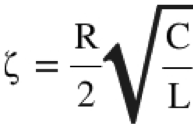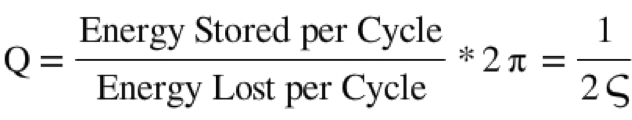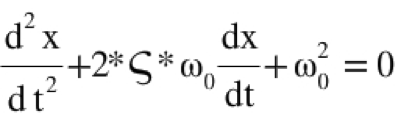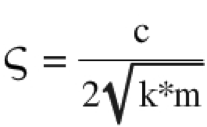# Q Factor and its Relevance in Electrical Circuits

By Jairam Sankar

33078

## Q factor and Damping Ratio

The damping ratio is a parameter, usually denoted by ζ (zeta), that characterizes the frequency response of a second order ordinary differential equation. It is particularly important in the study of control theory. It is also important in the harmonic oscillator.

The damping ratio provides a mathematical means of expressing the level of damping in a system relative to critical damping. The various expressions for damping ratio is given below:

### Damping Factor in an RLC Circuitwhere:

= Damping Factor

R = Resistance (Ω)

C = Capacitance (Fa)

L = Inductance (H)

### Q Factorwhere:

Q = Q Factor

= Damping Ratio

### Equation for Damped Harmonic Oscillatorwhere:

x = Position (m)

t = Time (s)

= Damping Ratio

### Damping Ratiowhere:

= Damping Ratio

c = Damping Coefficient

k = Spring Constant (N/m)

m = Mass (kg)

### Different Damped systems based on Q Factor

1. A system withlow quality factor (Q < 12) is said to be overdamped. Such a system doesn’t oscillate at all, but when displaced from its equilibrium steady-state output it returns to it by exponential decay, approaching the steady state value asymptotically.
2. A system withhigh quality factor (Q > 12) is said to be underdamped. Underdamped systems combine oscillation at a specific frequency with a decay of the amplitude of the signal. Underdamped systems with a low quality factor (a little above Q = 12) may oscillate only once or a few times before dying out.
3. A system with anintermediate quality factor (Q = 12) is said to be critically damped. Like an overdamped system, the output does not oscillate, and does not overshoot its steady-state output

When choosing defining the Q factor for a system, it is common to opt for the highest level. In this way the optimum performance is normally achieved. However there are instances where lower levels of Q may be advantageous.

I am currently pursuing my final year Btech in ECE at RSET, Cochin

1.Harvir

The definitions are incorrectly defined here.
The correct ones are:
Damping factor ( represented by alpha or Xi) = R/2L
Damping ratio ( represented by zeta) = Damping Factor/ Resonant Frequency.

The formula that you have mentioned under Damping Factor is actually for Damping Ratio.
Kindly do the needful.

•Nancy

What are the units of electrical damped oscillator the quality R/2L ?